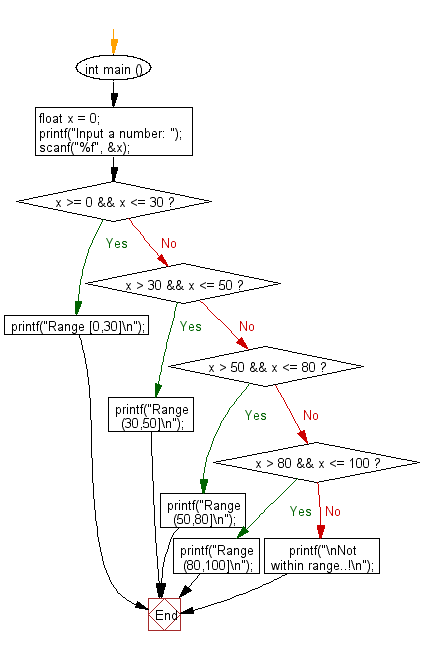﻿ C : Find the range of a floating-point number from 4 ranges

# C Exercises: Read a floating-point number and find the range where it belongs from four given ranges

## C Basic Declarations and Expressions: Exercise-101 with Solution

There are three given ranges. Write a C program that reads a floating-point number and finds the range where it belongs from four given ranges.

Ranges: 0-30, 31-50, 51-80, 81-100

Sample Solution:

C Code:

``````#include <stdio.h>

int main ()
{
// Declare a variable "x" to hold the input number
float x = 0;

// Prompt the user to input a number
printf("Input a number: ");

scanf("%f", &x);

// Check the range of the input number and print the corresponding message
if (x >= 0 && x <= 30)
printf("Range [0,30]\n");
else if (x > 30 && x <= 50)
printf("Range (30,50]\n");
else if (x > 50 && x <= 80)
printf("Range (50,80]\n");
else if (x > 80 && x <= 100)
printf("Range (80,100]\n");
else
printf("\nNot within range..!\n");
}
``````

Sample Output:

```Input a number: 87
Range (80,100]
```

Flowchart:C programming Code Editor:

What is the difficulty level of this exercise?

Test your Programming skills with w3resource's quiz.

﻿